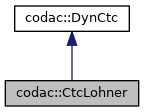codac
codac::CtcLohner Class Reference

$\mathcal{C}_\textrm{lohner}$ that contracts a tube $[\mathbf{x}](\cdot)$ according to a differential constraint $\dot{\mathbf{x}}=\mathbf{f}(\mathbf{x})$ More...

#include <codac_CtcLohner.h>

Inheritance diagram for codac::CtcLohner:[legend]
Collaboration diagram for codac::CtcLohner:[legend]

## Public Member Functions

CtcLohner (const Function &f, int contractions=5, double eps=0.1)
Creates a contractor object $\mathcal{C}_\textrm{lohner}$. More...

void contract (codac::TubeVector &tube, TimePropag t_propa=TimePropag::FORWARD|TimePropag::BACKWARD)
Contracts the tube with respect to the specified differential constraint, either forward, backward (or both) in time. More...

void contract (codac::Tube &tube, TimePropag t_propa=TimePropag::FORWARD|TimePropag::BACKWARD)
Contracts the tube with respect to the specified differential constraint, either forward, backward (or both) in time. More...Public Member Functions inherited from codac::DynCtc
DynCtc (bool intertemporal=true)
Creates a contractor. More...

virtual ~DynCtc ()
DynCtc destructor.

void preserve_slicing (bool preserve=true)
Specifies whether the contractor can impact the tube's slicing or not. More...

void set_fast_mode (bool fast_mode=true)
Specifies an optional fast mode of contraction. More...

void restrict_tdomain (const Interval &tdomain)
Limits the temporal domain of contractions. More...

bool is_intertemporal () const
Tests if the related constraint is inter-temporal or not. More...

## Protected Attributes

Function m_f
forward function

int contractions
number of contractions of the global enclosure by the estimated local enclosure

int dim
dimension of the state vector

double eps
inflation parameter for the global enclosureProtected Attributes inherited from codac::DynCtc
bool m_preserve_slicing = true
if true, tube's slicing will not be affected by the contractor

bool m_fast_mode = false
some contractors may propose more pessimistic but faster execution modes

Interval m_restricted_tdomain
limits the contractions to the specified temporal domain

const bool m_intertemporal = true
defines if the related constraint is inter-temporal or not (true by default)

## Static Protected Attributes

static const std::string m_ctc_name
class name (mainly used for CN Exceptions)

static std::vector< std::string > m_str_expected_doms
allowed domains signatures (mainly used for CN Exceptions)

## Detailed Description

$\mathcal{C}_\textrm{lohner}$ that contracts a tube $[\mathbf{x}](\cdot)$ according to a differential constraint $\dot{\mathbf{x}}=\mathbf{f}(\mathbf{x})$

## ◆ CtcLohner()

 codac::CtcLohner::CtcLohner ( const Function & f, int contractions = 5, double eps = 0.1 )
explicit

Creates a contractor object $\mathcal{C}_\textrm{lohner}$.

Parameters
 f function corresponding to the differential constraint $\dot{\mathbf{x}}=\mathbf{f}(\mathbf{x})$ contractions number of contractions of the global enclosure by the estimated local enclosure eps inflation parameter for the global enclosure

## ◆ contract() [1/2]

 void codac::CtcLohner::contract ( codac::TubeVector & tube, TimePropag t_propa = TimePropag::FORWARD|TimePropag::BACKWARD )

Contracts the tube with respect to the specified differential constraint, either forward, backward (or both) in time.

Parameters
 tube tube to contract t_propa direction of contraction

## ◆ contract() [2/2]

 void codac::CtcLohner::contract ( codac::Tube & tube, TimePropag t_propa = TimePropag::FORWARD|TimePropag::BACKWARD )

Contracts the tube with respect to the specified differential constraint, either forward, backward (or both) in time.

Parameters
 tube tube to contract t_propa direction of contraction

The documentation for this class was generated from the following file: